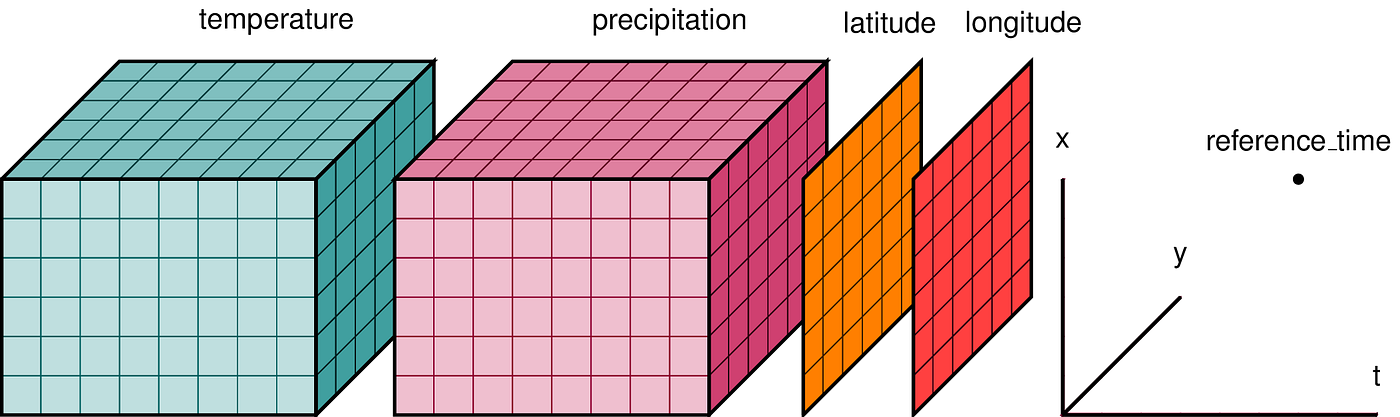# xframe, towards a C++ dataframeIn xframe and xarray, the base variable type is a labeled N-dimensional array (Illustration from the xarray documentation)

# Labeled arrays

`using coordinate_type = xf::xcoordinate<xf::fstring>;using variable_type = xf::xvariable<double, coordinate_type>;auto v = variable_type(    xt::eval(xt::random::rand({6, 3}, 15., 25.)),    {        {"group", xf::axis({"a", "b", "c", "d", "e", "f"})},        {"city", xf::axis({"NYC", "London", "Paris"})}    });`
`// Dimension and index lookup by positionv(0, 2);// Dimension lookup by position, index lookup by labelv.locate("a", "Paris");// Dimension lookup by name, index lookup by positionv.iselect({{"city", 2},           {"group", 0}});// Dimension lookup by name, index lookup by labelv.select({{"city", "Paris"},          {"group", "a"}});`
`auto v2 = variable_type(    {0.5, 0.7, 0.6, 0.3, 0.2, 0.6},    {{"group", xf::axis({"a", "b", "c", "d", "e", "f"})}});variable_type res = v1 + v2;`

# Zero-copy operations

`auto view = xf::select(v, {{"city", xf::keep("Paris")},                           {"group", xf::drop("a", "f")}});view.locate("b") = 0.;std::cout << v.locate("b", "Paris") << std::endl;// Prints 0.`